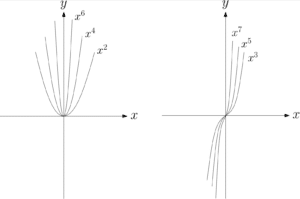# Curve Sketching

## Approach 1 - Steps for Curve Sketching

Follow these steps for sketching a curve:

1. Firstly, identify the general shape of the curve and whether it is of a negative or positive shape.
2. Next, find the y-intercept – substitute $x=0$ into the equation of the graph to see where the graph cuts the $y$-axis.
3. Then, identify the roots of the cubic – this is where the graph should cut the $x$-axis. This may involve factorising and you should note that the graph will bounce off the $x$-axis at any repeated roots.
4. Finally, place the curve so that it cuts the $x$ and $y$ axes at the correct points making sure that the curve touches the $x$ axis at any repeated roots.

See Example 1.

## Approach 2 - Applying Transformations to a known curve

Alternative to the above approach you may be asked to sketch a curve by performing transformations to a curve you already know or one that is given to you. Click here to see the various Transformations that you should know how to perform.

### Polynomials

You should know how to sketch some polynomials including Quadratics (see Quadratics or Completing the Square), Cubics (click here to see Cubic Sketching) and some Quartics (see above). Note that the shapes of the basic polynomials are as follows but they can each have central stationary points accordingly:See Completing the Square to see how to use Transformations to sketch a quadratic.

### Reciprocals

Reciprocals are curves that have asymptotes (lines that are approached but never touched) due to division by x, see example below. Note that curves with equation $y=\frac{1}{x-a}+b$ have horizontal and vertical asymptotes. These asymptotes have equations $x=-a$ (vertical) and $y=b$ (horizontal). You may be required to know the following graphs and to perform transformations to them. Both curves have asymptotes at $x=0$ and $y=0$.

See Example 2.

## Examples of Curve Sketching

Sketch the quartic $y=x^2(2x-1)^2$.

Sketch the graph of $y=\frac{2}{x-5}-3$.

## What Next?

For more on curve sketching:

Or: